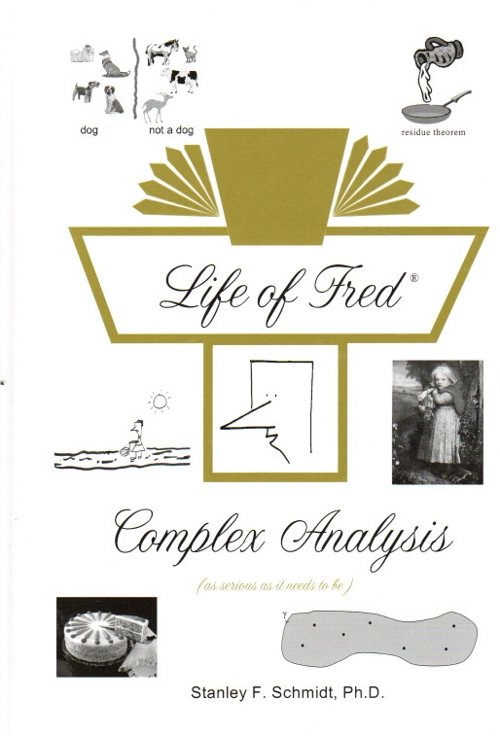Life of Fred Complex Analysis \$49 : Arithmetic in the complex plane. Conjugates and absolute value. Polar form. Complex functions. Graphing. Limits and derivatives. Cauchy-Riemann equations. Holomorphic functions. Regions, open sets, connected sets. Paths. Analytic functions. Integration along a path. Fundamental Theorem of Complex Integration. Piecewise smooth paths. Paths with corners or cusps. Cauchy’s theorem. Closed paths. Simple paths. Cauchy–Goursat theorem. Trig functions, exponents, and logs in the complex plane. Argument of a polar complex function. Morera’s theorem. Three kinds of isolated singular points. Order of a pole. Proving L’Hospital’s rule in the complex plane. Meromorphic functions. Casorati-Weierstrass theorem. Picard’s great theorem. Cauchy’s formula. Jordan curve theorem. Liouville theorem. Proof of the Fundamental Theorem of Algebra. Taylor series and Laurent series. Residue theorem. Analytic continuation. Riemann Zeta function. Conformal Mappings. Homotopic paths. Harmonic functions. ISBN: 978-1-937032-61-6, hardback, 176 pages. \$49